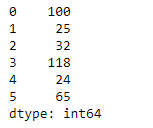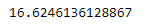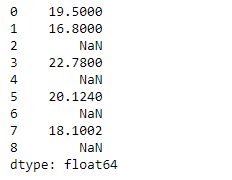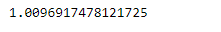Skip to content
Related Articles
Python | Pandas Series.sem()
• Last Updated : 05 Feb, 2019

Pandas series is a One-dimensional ndarray with axis labels. The labels need not be unique but must be a hashable type. The object supports both integer- and label-based indexing and provides a host of methods for performing operations involving the index.

Pandas` Series.sem()` function return unbiased standard error of the mean over requested axis. The result is normalized by N-1 by default. This can be changed using the ddof argument.

Syntax: Series.sem(axis=None, skipna=None, level=None, ddof=1, numeric_only=None, **kwargs)

Parameter :
axis : {index (0)}
skipna : Exclude NA/null values.
level : If the axis is a MultiIndex (hierarchical), count along a particular level, collapsing into a scalar.
ddof : Delta Degrees of Freedom.
numeric_only : Include only float, int, boolean columns.

Returns : scalar or Series (if level specified)

Example #1 : Use `Series.sem()` function to find the standard error of the mean of the underlying data in the given Series object.

 `# importing pandas as pd``import` `pandas as pd`` ` `# Creating the Series``sr ``=` `pd.Series([``100``, ``25``, ``32``, ``118``, ``24``, ``65``])`` ` `# Print the series``print``(sr)`

Output :Now we will use `Series.sem()` function to find the standard error of the mean of the underlying data.

 `# find standard error of the mean``sr.sem()`

Output :As we can see in the output, `Series.sem()` function has successfully calculated the standard error the mean of the underlying data in the given Series object.

Example #2 : Use `Series.sem()` function to find the standard error of the mean of the underlying data in the given Series object. The given Series object also contains some missing values.

 `# importing pandas as pd``import` `pandas as pd`` ` `# Creating the Series``sr ``=` `pd.Series([``19.5``, ``16.8``, ``None``, ``22.78``, ``None``, ``20.124``, ``None``, ``18.1002``, ``None``])`` ` `# Print the series``print``(sr)`

Output :Now we will use `Series.sem()` function to find the standard error of the mean of the underlying data.

 `# find standard error of the mean``# Skip all the missing values``sr.sem(skipna ``=` `True``)`

Output :As we can see in the output, `Series.sem()` function has successfully calculated the standard error the mean of the underlying data in the given Series object.

Attention geek! Strengthen your foundations with the Python Programming Foundation Course and learn the basics.

To begin with, your interview preparations Enhance your Data Structures concepts with the Python DS Course. And to begin with your Machine Learning Journey, join the Machine Learning – Basic Level Course

My Personal Notes arrow_drop_up